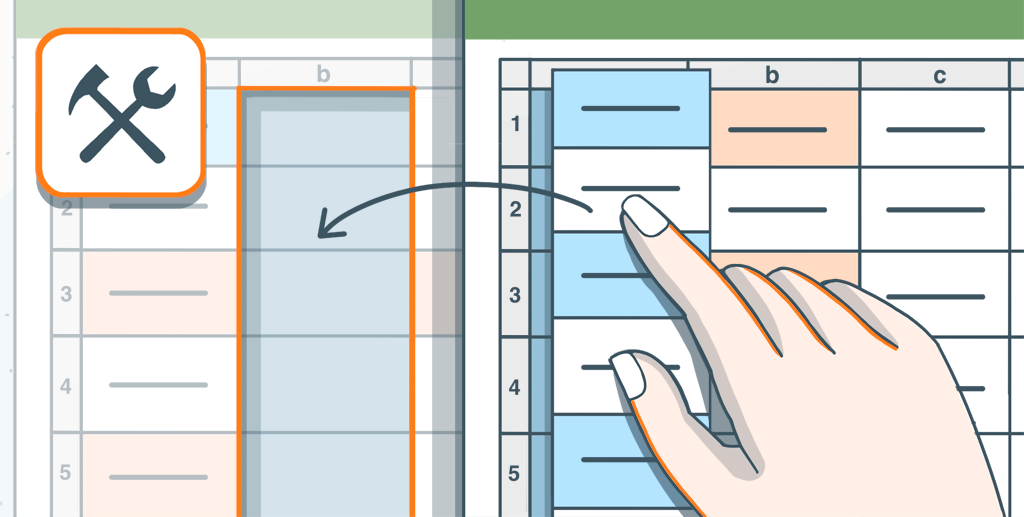# Use ONLYOFFICE macro to copy spreadsheets

Originally published at: Use ONLYOFFICE macro to copy spreadsheets | ONLYOFFICE Blog

Editing spreadsheets can be tricky, especially when handling multiple spreadsheets with similar data. To ease your process, let’s create a macro that copies data from one spreadsheet to another, and helps you handle large spreadsheets easily.## Building the macro

First, we access the current sheet in the editor. Then we access the sheet we want to copy to in the pastesheet variable, following the oRange variable which specifies the range we want to work on.

```  const oWorksheet = Api.GetActiveSheet();
const pastesheet = Api.GetSheet("Sheet2");
const oRange = oWorksheet.GetRange("A1:C10");
// If you want to copy a specific Selection to the new sheet
// var oRange = oWorksheet.GetSelection();
```

Note: If you want to work on a selection, use the GetSelection() method instead by uncommenting the line above.

Next, we use the for each method on the specified range, to iterate through each cell in the range.

```oRange.ForEach(function (range) {
```

Using the parameter range in the callback function, we get access to the data of each cell, starting from the first cell.

```  const oValue = range.GetValue();
const oCharacters = range.GetCharacters(0, 2);
const oFont = oCharacters.GetFont();
const fontName = oFont.GetName();
const oSize = oFont.GetSize();
const isBold = oFont.GetBold();
const isItalic = oFont.GetItalic();
```

In the ForEach method, first we extract the value of the cell. Next we extract the initial characters of the value to know its parameters like boldness, italics and etc. Then we get the fontName, followed by the font size, in order to implement them in the new sheet.

```if (oValue === null || oValue === "") {
pastesheet.GetRange(range).SetValue(" ");
}
```

Before putting the values in the new sheet, we run some checks using if else loops to make sure empty cells are represented correctly in the new sheet, as shown above.

```else {
oFont.SetName(fontName);
pastesheet.GetRange(range).SetValue(oValue);
pastesheet.GetRange(range).SetFontName(fontName);
pastesheet.GetRange(range).SetFontSize(oSize);
oWorksheet.GetRange(range).AutoFit(false, true);
if (isBold) {
pastesheet.GetRange(range).SetBold(true);
}
if (isItalic) {
pastesheet.GetRange(range).SetItalic(true);
}
}
```

Finally in the else loop, we put the values in their corresponding cells, and then give those cells their properties like boldness, italics, fontname and font size.

## The full macro

Here is the entire macro code:

```(function () {
const oWorksheet = Api.GetActiveSheet();
const pastesheet = Api.GetSheet("Sheet2");
const oRange = oWorksheet.GetRange("A1:C10");
// If you want to copy a specific Selection to the new sheet
// var oRange = oWorksheet.GetSelection();
oRange.ForEach(function (range) {
const oValue = range.GetValue();
const oCharacters = range.GetCharacters(0, 2);
const oFont = oCharacters.GetFont();
const fontName = oFont.GetName();
const oSize = oFont.GetSize();
const isBold = oFont.GetBold();
const isItalic = oFont.GetItalic();
if (oValue === null || oValue === “”) {
pastesheet.GetRange(range).SetValue(" ");
} else {
oFont.SetName(fontName);
pastesheet.GetRange(range).SetValue(oValue);
pastesheet.GetRange(range).SetFontName(fontName);
pastesheet.GetRange(range).SetFontSize(oSize);
oWorksheet.GetRange(range).AutoFit(false, true);
```  if (isBold) {
pastesheet.GetRange(range).SetBold(true);
}
if (isItalic) {
pastesheet.GetRange(range).SetItalic(true);
}
}
```
});
})();
```

Some key points to remember:

1. Make sure the sheet name you specify in the macro exists, and is preferably a blank sheet.
2. Make sure to run the macro from the main sheet (the parent sheet), and not the newly created sheet.

Let’s run our macro and see how it works!

Here is another example of the macro working with the GetSelection() method: